407 493 6601

# 7th Grade - Graphs and Charts

## Introduction

• To draw meaningful inferences from a given data set, we need to organize the data systematically and then display it visually.
• Line plots, box plots, histograms, stem-and-leaf plots, and circle plots are some ways to visualize data for easier analysis of the data set.

## Line Plots

• A line plot is a way to graphically display a given data set on a number line.
• The horizontal line (number line) shows the categories being considered.
• The frequency corresponding to each category is displayed using dots, crosses, or any other symbol over them.
• In the line plot shown below, the number line displays the time taken by students to complete a test (in minutes) and the "circle" mark represents the number of students (frequency).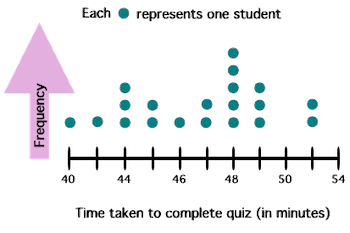## Box Plots

• A box plot is a way to display the distribution of a given data set.
• Box plot displays five key parameters about a data set as mentioned below:
• the minimum value,
• the first quartile $\left({Q}_{1}\right)$,
• median,
• the third quartile $\left({Q}_{3}\right)$, and
• the maximum value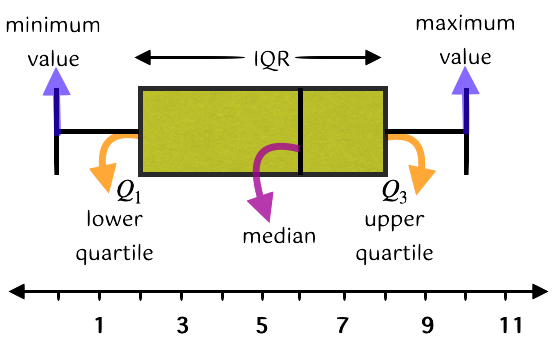• We can also compute the range and interquartile range (IQR) of a given data set from the box plot.
• Range:
• IQR: ${Q}_{3}-{Q}_{1}$

## Histograms

• A histogram is a graphical display of continuous numerical data distribution (just like bar graphs are used to represent categorical data).
• The horizontal axis of a histogram represents the class interval (bins) while the height of each bin displays the frequency of data values in that interval.
• Recall that frequency means the number of times a particular entry occurs.
• The histogram shown below displays the weekly self-study duration of some of the students in Grade 7.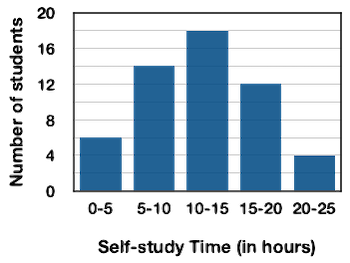• It should be noted that the width of the bins is equal.
• A histogram also displays whether the distribution is skewed or symmetric.

## Stem-and-Leaf Plot

• A stem and leaf plot is a way to organize and display data in a special table.
• Each data value is broken into a stem and a leaf.
• The 'stem' is on the left side of the table & displays the first digit or digits of the data.
• The 'leaf' is on the right side of the table & displays the last digit.
• The stem and leaf plot key at the bottom of the table helps us understand the data values.
• If we combine the values of the stem and the leaves (as per the rule indicated by the key), we will get the data values.
• For example, the stem and leaf plot shown below represents the age of people who watched a particular movie on Sunday in City Mall.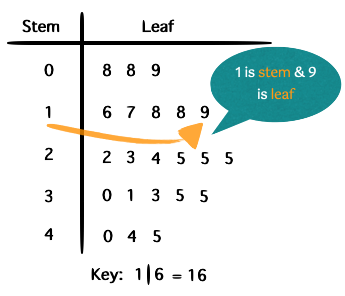## Circle Graph (Pie Chart)

• A circle graph aka Pie Chart is used to visualize data (as a fractional part of a whole) on a circle.
• In a circle graph, the area of a circle is divided into slices to display the numerical proportion of each category.
• Each angle of the circle graph is proportional to the quantity it represents.
• Percentage of a category =
• The angle subtended by a category =
• The pie chart shown below exhibits the distribution of favorite games of the population of residents of New York City.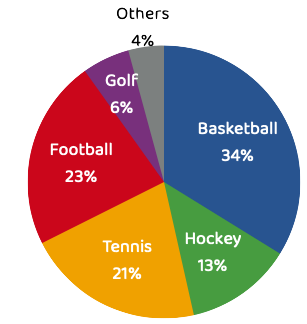## Solved Examples

Question 1: The line plot shown below represents the time taken by some of the university students to complete a bike race. Find the mean and median time taken to complete the race.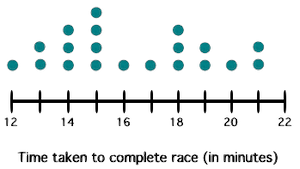Solution:

$=\frac{\left(12×1\right)+\left(13×2\right)+\left(14×3\right)+\left(15×4\right)+\left(16×1\right)+\left(17×1\right)+\left(18×3\right)+\left(19×2\right)+\left(20×1\right)+\left(21×2\right)}{\left(1+2+3+4+1+1+3+2+1+2\right)}$

$=\frac{327}{20}$

Median = value of middlemost observation after arranging the data in ascending/descending order

There are 20 data values. The Median will be the average of ${10}^{th}$ & ${11}^{th}$ value.

Question 2: The stem-and-leaf plot shown below represents the scores of 25 students on a test (out of 50 marks). Find the mode score.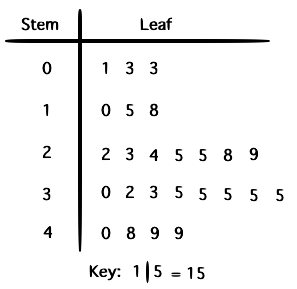Solution: Mode is the data that occurs most frequently in a given data set. It can be clearly seen from the stem-and-leaf plot that a score of 35 occurs most frequently (6 times), and hence 35 is the mode score.

Question 3: The box plot shown below represents the duration (in hours) of TV watched by some students in a given month. Determine the median of watch time.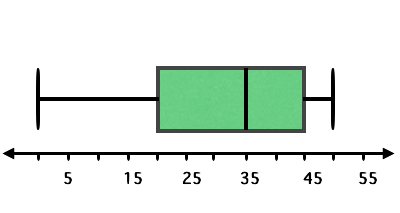Solution: Median = value of middlemost observation after arranging the data in ascending/descending order

From the given plot, it is clearly evident that the median watch time is 35 hours.

Question 4: The marks scored by 100 students of Grade 7 in the Mathematics test (out of 50) was recorded and displayed in the histogram shown below. If a student scores 20 and above, he is declared as 'qualified'. Find the number of students who did not qualify for the test.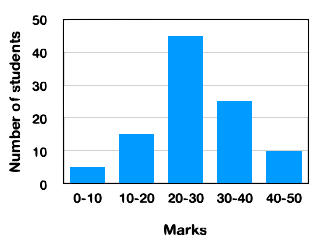Solution: From the histogram shown above, the number of students who could not qualify for the test = 5 + 15 = 20.

Question 5: The circle chart shown below represents the expenditure on various items by an international student in Florida. If his total monthly expenditure is $1,900, find the money spent on food.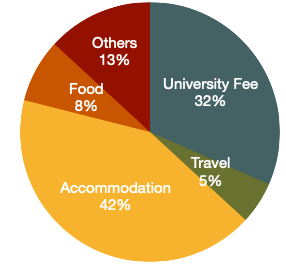Solution: Total monthly expenditure =$1,900.

Expenditure on food = $=\frac{8}{100}×1,900$$=152$

## Cheat Sheet

• A line plot is drawn on a number line that represents categories and the frequency of data points corresponding to each category is displayed above it using dots, crosses, or any other symbol.
• Through a box plot, we get information regarding 5 key parameters of a dataset - the minimum value, first quartile, median, third quartile, and maximum value.
• A histogram is a type of bar graph, where the class intervals are shown on the horizontal axis and the heights of the bars display the frequency of the class interval.
• In a stem-and-leaf plot, each data point is split into two components - a stem and a leaf. Combining the satem and leaf data using a predefined key gives back the original data point.
• A circle graph shows the relationship between a whole and its part.

## Blunder Areas

• It is good practice to identify outliers before drawing charts. This is because outliers can have a disproportionate effect on the statistical results (the results can be misleading).